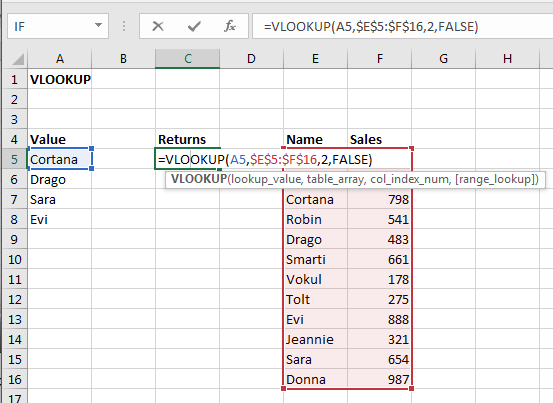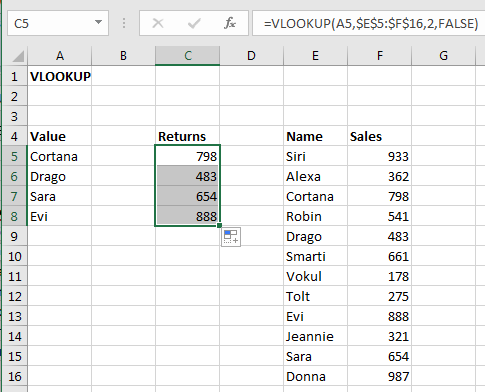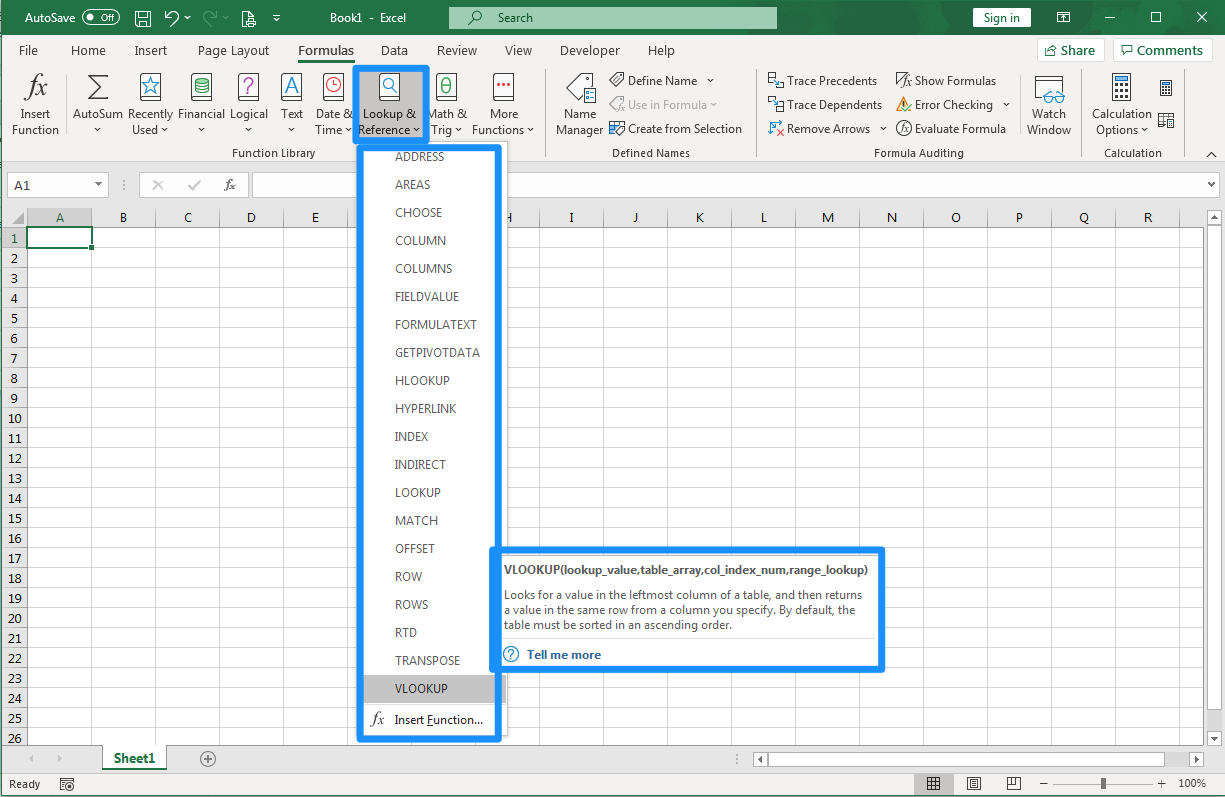## Lookup Functions and Formulas

### Learning Outcomes

• Use lookup functions and formulas

Lookup functions in Excel are used for looking through a single column or row to find a particular value from the same place in a second column or row. This often takes place when there are multiple worksheets within a workbook or a large amount of data in a worksheet. Lookup functions are very helpful with large sets of data to find specific information across many rows or columns.

## VLOOKUP (Vertical Lookup)

Looks for a value in the leftmost column of a table and returns a value in the same row from the vertical column you define. The lookup value must always be in the leftmost column of the table.

The Excel formula is written =VLOOKUP(lookup_value,table_array,col_index_num,[range_lookup])

This table includes the sales totals for salespeople. In this case you want to find out what the sales were for certain salespeople. This is a small example but imagine a long list of names and numbers to search through. Using the VLOOKUP function quickly brings you the results you want. Be sure to use F4 to create the absolute references and keep the table array the same for each lookup. Also make sure and make the col_index_num use the second column in the table (column 2 in the table) with a return for the range_lookup as FALSE. By using FALSE in all cases, there is no need to sort your tables into ascending order. Here is what those results look like.For another VLOOKUP example, watch this video of how to use this function to find parts that are in stock and the supplier for particular parts.

## HLOOKUP (Horizontal Lookup)

Looks for a value in the topmost row of a table and returns a value in the same row from the horizontal column you define. The lookup value must always be in the leftmost column of the table.

The Excel formula is written =HLOOKUP(lookup_value,table_array,row_index_num,[range_lookup])

## MATCH and INDEX Functions

Now we’ll learn two additional lookup functions, Match and Index. Watch this video to learn how to use Match and Index lookup functions. Here are the descriptions and formulas for creating them in Excel.

• MATCH – Function that returns a position of a value in a range which can be a row, column or table. The Excel formula is written =MATCH(lookup_value,lookup_array,[match_type])
• INDEX – Function that returns a position of a value in a range which looks at the intersection of a column and row position in a table. The Excel formula is written =INDEX(reference,row_num,[column_num],[area_num])

As you become more familiar with lookup functions, try out a few more by selecting the Formulas tab, Lookup & Reference button and see what others are available for you to try.## Contribute!

Did you have an idea for improving this content? We’d love your input.